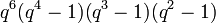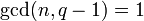# Element structure of special linear group over a finite field

This article gives specific information, namely, element structure, about a family of groups, namely: special linear group.
View element structure of group families | View other specific information about special linear group

## Particular cases

### Particular cases by degree

Value of degree$n$ Element structure of
special linear group$SL(n,q)$
order of group Degree as a polynomial in$q$ (=$n^2 - 1$) Number of conjugacy classes (see number of conjugacy classes in special linear group of fixed degree over a finite field is PORC function of field size)
1 it is the trivial group 1 0 1
2 link$q^3 - q$ 3$q + 1$ if$q$ even$q + 4$ if$q$ odd
3 link$q^3(q^3 - 1)(q^2 - 1)$ 8$q(q + 1)$ if$q$ not 1 mod 3$q^2 + q + 8$ if$q$ is 1 mod 3
4 link$q^6(q^4 - 1)(q^3 - 1)(q^2 - 1)$ 15$q^3 + q^2 + q$ if$q$ even$q^3 + q^2 + 4q + 3$ if$q$ is 3 mod 4$q^3 + q^2 + 4q + 15$ if$q$ is 1 mod 4

## Conjugacy class structure

### Strategy

The strategy for determining the conjugacy class structure involves two steps:

1. First, we identify the$GL(n,q)$-conjugacy classes of elements that are in$SL(n,q)$.$SL(n,q)$ is a normal subgroup of$GL(n,q)$, so every$GL(n,q)$-conjugacy class is either completely inside or completely outside$SL(n,q)$.
2. Then, we use the splitting criterion for conjugacy classes in the special linear group to figure out, for each conjugacy class, whether it splits, and if so, how many pieces it splits into.

## Finding the GL-conjugacy classes

The execution of step (1) (identifying the$GL(n,q)$-conjugacy classes in$SL(n,q)$) relies on the same ideas as those behind element structure of general linear group over a finite field, but while computing the number of conjugacy classes of each type, we need to impose the additional constraint of determinant 1.

## Splitting criterion

### Splitting criterion: general rule

Further information: splitting criterion for conjugacy classes in the special linear group

We now need to execute step (2), i.e., figure out which conjugacy classes split, and how much. The general rule is as follows: for an element$g \in SL(n,q)$, the$GL(n,q)$-conjugacy class of$g$ does not split if and only if the image of$C_{GL(n,q)}(g)$ under the determinant map is all of$\mathbb{F}_q^\ast$. Further, if it does split, the number of pieces it splits into is the quotient group of$\mathbb{F}_q^\ast$ by the image of$C_{GL(n,q)}(g)$ under the determinant map

### Results specific to finite fields

It turns out that, since we are working over a finite field, we have the fact that the algebraic norm map is surjective, which indirectly guarantees that a lot of conjugacy classes do not split. More explicitly, we have the following: conjugacy class of elements with semisimple generalized Jordan block does not split in special linear group over a finite field. In other words, the only conjugacy classes that could potentially split in the special linear group are those in which all the generalized Jordan blocks have multiplicity at least two. Even among these, it is possible that the conjugacy class ultimately does not split -- figuring this out requires a calculation of the image of the determinant map.

### Case of prime degree

For simplicity, we consider the case where the degree$n$ is a prime number (not necessarily the characteristic of the field, just any prime number). In this case, it turns out that the following is true:

• If$\operatorname{gcd}(n,q - 1) = 1$, then none of the conjugacy classes split (in fact, in this case,$SL(n,q)$ is a direct factor of$GL(n,q)$, hence a conjugacy-closed subgroup; for more, see isomorphism between linear groups when degree power map is bijective).
• If$\operatorname{gcd}(n,q - 1) = n$, then the only conjugacy classes that split are the ones that comprise a single Jordan block of size$n$. There are$n$ such$GL(n,q)$-conjugacy classes, and each one splits into$n$ conjugacy classes in$SL(n,q)$, so there is a total of$n^2$ such conjugacy classes in$SL(n,q)$.

Some small examples of values of$n$ are$n = 2$ (see element structure of special linear group of degree two over a finite field) and$n = 3$ (see element structure of special linear group of degree three over a finite field).